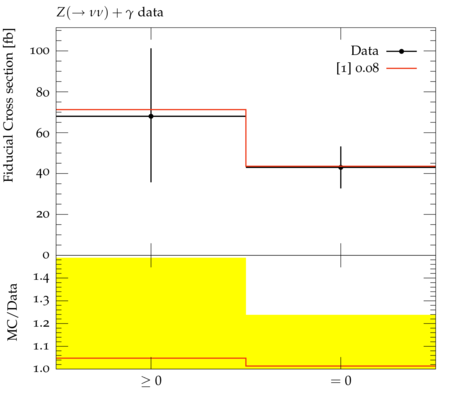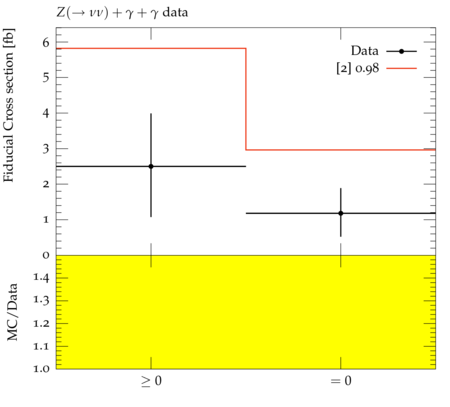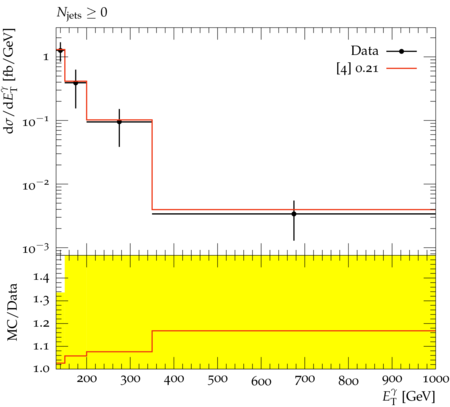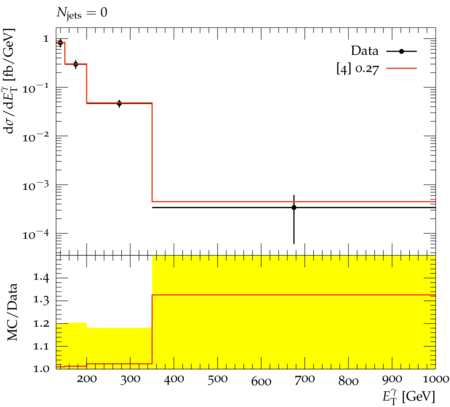### ATLAS_2016_I1448301_NU

Back to index

The production of $Z$ bosons with one or two isolated high-energy photons is studied using pp collisions at $\sqrt{s}=8$ TeV. The analyses use a data sample with an integrated luminosity of 20.3 fb${}^{-1}$ collected by the ATLAS detector during the 2012 LHC data taking. The $Z\gamma$ and $Z\gamma\gamma$ production cross sections are measured with leptonic ($e^+e^-$, $\mu^+\mu^-$, $\nu\bar{\nu}$) decays of the Z boson, in extended fiducial regions defined in terms of the lepton and photon acceptance. They are then compared to cross-section predictions from the Standard Model, where the sources of the photons are radiation off initial-state quarks and radiative Z-boson decay to charged leptons, and from fragmentation of final-state quarks and gluons into photons. The yields of events with photon transverse energy $E_\text{T}>250$ GeV from $\ell^+\ell^-\gamma$ events and with $E_\text{T}>400$ GeV from $\nu\bar{\nu}\gamma$ events are used to search for anomalous triple gauge-boson couplings $ZZ\gamma$ and $Z\gamma\gamma$. The yields of events with diphoton invariant mass $m_{\gamma\gamma}>200$ GeV from $\ell^+\ell^-\gamma\gamma$ events and with $m_{\gamma\gamma}>300$ GeV from $\nu\bar{\nu}\gamma\gamma$ events are used to search for anomalous quartic gauge-boson couplings $ZZ\gamma\gamma$ and $Z\gamma\gamma\gamma$. No deviations from Standard Model predictions are observed and limits are placed on parameters used to describe anomalous triple and quartic gauge-boson couplings. The lepton channel can be specified by using the relevant plugins (_EL, _MU, _NU). The default plugin uses the electron channel.

ATLAS_2016_I1448301_NU_d02-x01-y01:ATLAS_2016_I1448301_NU_d04-x01-y01:ATLAS_2016_I1448301_NU_d07-x01-y01:ATLAS_2016_I1448301_NU_d08-x01-y01:Generated at Sunday, 28. January 2018 12:11PM